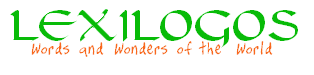a

b

'b

g

d

h

v

z

H

T

y

k

'k

l

m

n

s

j
ʿ

f

p

S
ts

q

r

c
sh/s

sh

s

t

ə

ĕ

ă

ŏ

ī

ē

é/ẹ

á/ạ

ā/ọ

ō

ū

cantillation marks

To switch the font:

Instructions

To type directly with the computer keyboard:

• Use the capitals: H for ח, T for ט, S for צ
• Type c for ש
• Type c or w for ש
• Type c= for and c== for
• Type a for א and type j for ע
• Type a space key to form the final characters: ex. m + [space key] for ם
• Type an apostrophe ['] to add the daguesh: ex. b' for
• Type a=, a==, a===, i=, i==, i===, u=, u==, u===, u==== to add a diacritic sign for a vowel
• Type x to add the meteg
• Type hataf (ĕ, ă, ŏ) + meteg, for a medially positioned; example : tăx תֲֽ (ă halaf patah: a===)
• Type hataf (ĕ, ă, ŏ) + L + meteg, for a Left-positioned; example: tăLx תֲ‌ֽ
• Type meteg + R + hataf (ĕ, ă, ŏ), for a Right-positioned; example: txRă תֽ͏ֲ
• Type diacritic + R + diacritic, to reverse the order of the diacritics (to place them Right to left).
• To get the furtive patah, use the font Ezra; example: vHá וּחַ

Copy [Ctrl]+[C] & Paste [Ctrl]+[V]